# Circular Queue in C | Circular Queue

In this article circular queue in c we give the information about we also call circular queue as ring-buffer. The last node in the circular queue is connected to the first node. So that the circle is formed.

#### Circular queue in C:-

We also call circular queue as ring-buffer. The last node in the circular queue is connected to the first node. So that the circle is formed. It works on the principle of FIFO. In Circular Queue, item is added from rear end and item is removed from front end.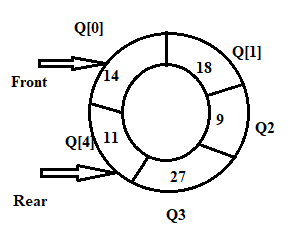A Circular queue overcomes the problem of unused space in linear queues. A circular queue also has front and rear to keep the track of the elements to be deleted and inserted. The following assumptions are made about circular queue:-

• Front always points the first elements in circular queue.
• Rear is incremented every time when a new element is to added.
• Front is incremented every time when an element is to deleted.
• If front = rear then queue is empty.

#### Operations on Circular queue:-

The following figures shows the add and delete operations on queue.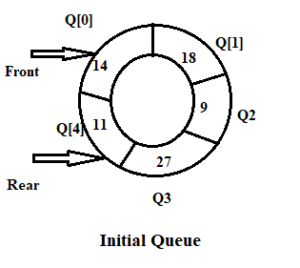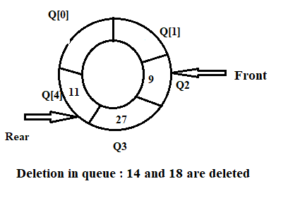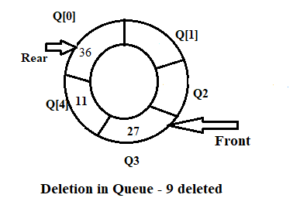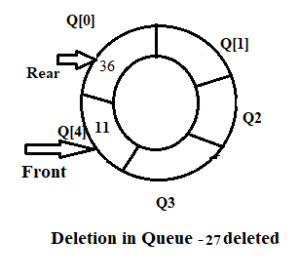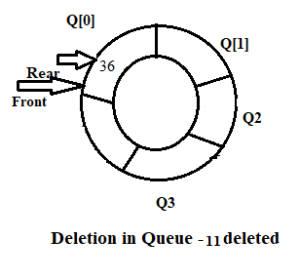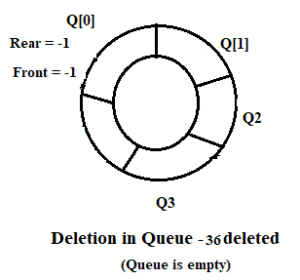There are two operations in circular queue

2. Delete operation on queue.

#### 1.Add Operation in Circular Queue:-

First check whether queue is full or not and then add an elements in queue.

If front =0 and rear =MAX-1 or front =rear +1 then queue is full

if((front==0 && rear == MAX-1) || (front == rear +1))

{

printf(“\n Queue is overflow…”);

return;

}

If queue is initially empty then set the value of front and rear to -1 and then add an element in queue. Otherwise increase the value of rear only and then add an element.

If queue is full and rear = MAX-1 then set the value of rear to 0 and add an element.

if(front==-1)

{

front = 0;

rear = 0;

}

else

if(rear == MAX – 1)

rear =0 ;

else

rear =rear +1;

data[rear]=element;

#### 2.Delete operation on queue:-

First check whether queue is empty or not and then delete an element from queue.

If front = -1 and rear =-1 then queue is said to be empty.

If front = MAX – 1 then delete an elements from queue and set the value of front = 0.

if(front == MAX-1)

front=0;

if there is only one element in queue then delete an element from queue and set the value of front and rear to -1.

if (front ==rear)

{

front = -1;

rear = -1;

}

#### Circular Queue Example:-

/* Implementation of Circular Queue
This program implements the insert and delete operation in circular queue */

#include<stdio.h>
#include<conio.h>
#define MAX 5
struct queue
{
int front,rear;
int data[MAX];
}q;
void main()
{
int n,ch; // variable declaration
void insert(int); // function declaration
void delete1();
void display();
q.front=q.rear=-1;
clrscr();
while(1)
{
printf(“\n1:Insert \n2:Delete \n3:Display \n4:Exit”);
scanf(“%d”,&ch);
switch(ch)
{
case 1:
printf(“\n Enter number to insert: “);
scanf(“%d”,&n);
insert(n);
break;
case 2:
delete1();
break;
case 3:
display();
break;
case 4:
exit(0);
default:
printf(“\n Enter correct choice…”);
break;
}
getch();
}
}
void insert(int no)
{
if((q.front==0 && q.rear==MAX-1) || (q.front==q.rear+1))
{
printf(“\n Queue Overflow…”);
}
else
{
if(q.front==-1)

q.front=q.rear=0;

else

if(q.rear==MAX-1)

q.rear=0;

else

q.rear=q.rear+1;
q.data[q.rear]=no;
printf(“\n %d is inserted into QUEUE…”,no);
}

}
void delete1()
{
int temp;
if(q.front==-1)
printf(“\n Queue is Empty…”);
else
{
temp=q.data[q.front];
printf(“\n %d is deleted…”,temp);
if(q.front==q.rear)
q.front=q.rear=-1;
else
if(q.front==MAX-1)
q.front=0;
else
q.front=q.front+1;
}
}
void display()
{
int i;
if(q.front==-1)
printf(“\n Queue is Empty”);
else
{
for(i=q.front;i<=q.rear;i++)
printf(“\n %d”,q.data[i]);
if(q.front>q.rear)
{
for(i=q.front;i<MAX;i++)
printf(“\t %d”,q.data[i]);
for(i=0;i<=q.rear;i++)
printf(“\t %d”,q.data[i]);
}
}
}

#### Some More:

POP- Introduction to Programming Using ‘C’

DS – Data structure Using C

OOP – Object Oriented Programming

Java Programming

DBMS – Database Management System

RDBMS – Relational Database Management System